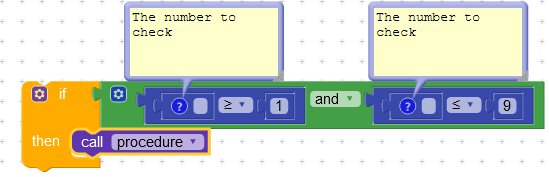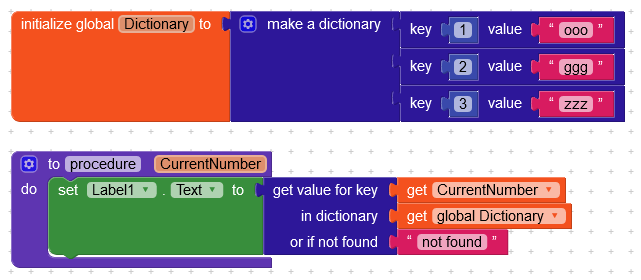# If the number is from 1 to 9, How to use this logic in block

I want to use this logic in block code.
If i get number from 1 to 9 then ( function)
It can be any number from 1 to 9.

Use this block -2 Likes

I want to function on each number I received

I didn’t get you…

See here -

ok… in my app i am going to receive number 1 to 60
and i want to function for each number i receive .
Eg: if i receive " 2 " then set variable = ooo
if i receive " 36 " then set variable = ggg
if i receive " 45 " then set variable = iooTry using such a block.
Call the procedure with a param, that will be the number received.

7 Likes